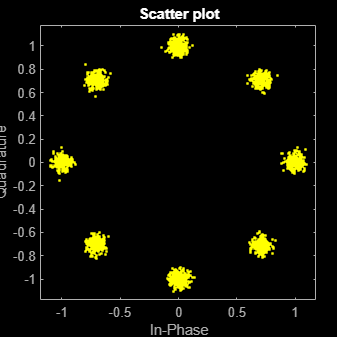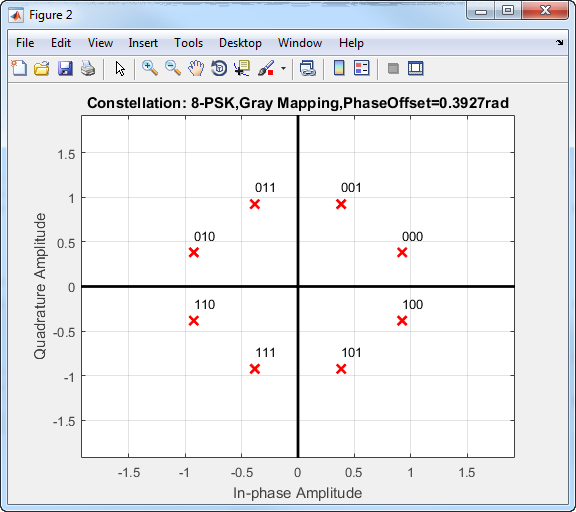# comm.PSKModulator

Modulate signal using M-PSK method

## Description

The `PSKModulator` System object™ modulates using the M-ary phase shift keying (M-PSK) method. The output is a baseband representation of the modulated signal.

To modulate a signal by using the M-PSK method:

1. Create the `comm.PSKModulator` object and set its properties.

2. Call the object with arguments, as if it were a function.

## Creation

### Syntax

``mpskmod = comm.PSKModulator``
``mpskmod = comm.PSKModulator(Name,Value)``
``mpskmod = comm.PSKModulator(M,phase,Name,Value)``

### Description

example

````mpskmod = comm.PSKModulator` creates a modulator System object `mpskmod`, that modulates the input signal using the M-ary phase shift keying (M-PSK) method.```
````mpskmod = comm.PSKModulator(Name,Value)` creates an M-PSK modulator object `mpskmod`, with each specified property set to the specified value. You can specify additional name-value pair arguments in any order as (`Name1`,`Value1`,...,`NameN`,`ValueN`).```
````mpskmod = comm.PSKModulator(M,phase,Name,Value)` creates a M-PSK modulator object `mpskmod` using the modulation order specified in `M`. The object's `PhaseOffset` property is set to `phase`, and the other specified properties are set to the specified values.```

## Properties

expand all

Unless otherwise indicated, properties are nontunable, which means you cannot change their values after calling the object. Objects lock when you call them, and the `release` function unlocks them.

If a property is tunable, you can change its value at any time.

Number of points in the signal constellation, specified as a positive, integer scalar.

Phase of the zeroth point of the constellation in radians, specified as a finite real-valued scalar.

Option to provide input in bits, specified as a numeric or logical `0` (`false`) or `1` (`true`).

• If you set this property to `0` (`false`), the input values must be integer representations of two-bit input segments and range between 0 to 3.

• If you set this property to `1` (`true`), the input must be a binary vector of even length. Element pairs are binary representations of integers.

Data Types: `logical` | `char`

Signal constellation bit mapping, specified as `'Gray'`, `'Binary'`, or `'Custom'`.

• `'Gray'` — Use this value to specify Gray-encoded signal constellation mapping.

• `'Binary'` — The integer m must be in the range [0, ModulationOrder – 1] and map to the complex value exp(j(PhaseOffset + 2πm/ModulationOrder))

• `'Custom'` — Use this value to specify the signal constellation mapping by using the CustomSymbolMapping property.

Data Types: `char` | `double`

Custom constellation encoding, specified as a row vector or column vector of values in the range [0, ModulationOrder – 1]. The length of this vector must be equal to the ModulationOrder property value. The first element of this vector corresponds to the constellation point at an angle of PhaseOffset, with subsequent elements running counterclockwise. The last element corresponds to the constellation point at an angle of – 2π/ModulationOrder + PhaseOffset.

#### Dependencies

To enable this property, set the SymbolMapping property to `'Custom'`.

Data Types: `double`

Output data type, specified as either `'double'`, `'single'` or `'Custom'`.

Fixed-Point Properties

Fixed-point data type of the output signal, specified as a `numerictype` object with its `Signedness` property set to `Auto`. To create this type of object, use the `numerictype` (Fixed-Point Designer) function.

#### Dependencies

To enable this property, set the OutputDataType property to `'Custom'`.

## Usage

### Syntax

``mpsksignal = mpskmod(insignal)``

### Description

````mpsksignal = mpskmod(insignal)` modulates the input signal by using the M-PSK method. The output is the modulated M-PSK baseband signal.```

### Input Arguments

expand all

Input signal, specified as a column vector of integers or bits. The vector must be of length NS, where NS is the number of samples.

The setting of the BitInput property determines the interpretation of the input vector.

Data Types: `double`

### Output Arguments

expand all

M-PSK modulated baseband signal, returned as a vector.

## Object Functions

To use an object function, specify the System object as the first input argument. For example, to release system resources of a System object named `obj`, use this syntax:

`release(obj)`

expand all

 `constellation` Calculate or plot ideal signal constellation
 `step` Run System object algorithm `release` Release resources and allow changes to System object property values and input characteristics `reset` Reset internal states of System object

## Examples

collapse all

Modulate an 8-PSK signal, add white Gaussian noise, and plot the signal to visualize the effects of the noise.

Create a M-PSK modulator System object™. The default modulation order for the object is 8.

`pskModulator = comm.PSKModulator;`

Modulate the signal.

`modData = pskModulator(randi([0 7],2000,1));`

Add white Gaussian noise to the modulated signal by passing the signal through an additive white Gaussian noise (AWGN) channel.

`channel = comm.AWGNChannel('EbNo',20,'BitsPerSymbol',3);`

Transmit the signal through the AWGN channel.

`channelOutput = channel(modData);`

Plot the noiseless and noisy data by using scatter plots to visualize the effects of the noise.

`scatterplot(modData)``scatterplot(channelOutput)`Change the `EbNo` property to 10 dB to increase the noise.

`channel.EbNo = 10;`

Pass the modulated data through the AWGN channel.

`channelOutput = channel(modData);`

Plot the channel output. You can see the effects of increased noise.

`scatterplot(channelOutput)`Create 16-PSK modulator and demodulator System objects™ which use custom symbol mapping. Estimate the BER in an AWGN channel and compare the performance to a theoretical Gray-coded PSK system.

Create a custom symbol mapping for the 16-PSK modulation scheme. The 16 integer symbols must have values which fall between 0 and 15.

`custMap = [0 2 4 6 8 10 12 14 15 13 11 9 7 5 3 1];`

Create a 16-PSK modulator and demodulator pair having custom symbol mapping defined by the array, `custMap`.

```pskModulator = comm.PSKModulator(16,'BitInput',true, ... 'SymbolMapping','Custom','CustomSymbolMapping',custMap); pskDemodulator = comm.PSKDemodulator(16,'BitOutput',true, ... 'SymbolMapping','Custom','CustomSymbolMapping',custMap);```

Display the modulator constellation.

`constellation(pskModulator)`Create an AWGN channel System object for use with 16-ary data.

`awgnChannel = comm.AWGNChannel('BitsPerSymbol',log2(16));`

Create an error rate object to track the BER statistics.

`errorRate = comm.ErrorRate;`

Initialize the simulation vectors. The ${\mathit{E}}_{\mathrm{b}}/{\mathit{N}}_{0}$ is varied from 6 to 18 dB in 1 dB steps.

```ebnoVec = 6:18; ber = zeros(size(ebnoVec));```

Estimate the BER by modulating binary data, passing it through an AWGN channel, demodulating the received signal, and collecting the error statistics.

```for k = 1:length(ebnoVec) % Reset the error counter for each Eb/No value reset(errorRate) % Reset the array used to collect the error statistics errVec = [0 0 0]; % Set the channel Eb/No awgnChannel.EbNo = ebnoVec(k); while errVec(2) < 200 && errVec(3) < 1e7 % Generate a 1000-symbol frame data = randi([0 1],4000,1); % Modulate the binary data modData = pskModulator(data); % Pass the modulated data through the AWGN channel rxSig = awgnChannel(modData); % Demodulate the received signal rxData = pskDemodulator(rxSig); % Collect the error statistics errVec = errorRate(data,rxData); end % Save the BER data ber(k) = errVec(1); end```

Generate theoretical BER data for an AWGN channel using the `berawgn` function.

`berTheory = berawgn(ebnoVec,'psk',16,'nondiff');`

Plot the simulated and theoretical results. The 16-PSK modulation BER performance of the simulated custom symbol mapping is not as good as the theoretical prediction curve for Gray codes.

```figure semilogy(ebnoVec,[ber; berTheory]) xlabel('Eb/No (dB)') ylabel('BER') grid legend('Simulation','Theory','location','ne')```## Algorithms

The block outputs a baseband signal by mapping input bits or integers to complex symbols according to the following:

`${s}_{n}\left(t\right)=\mathrm{exp}\left(j\pi \left(\frac{2n+1}{M}\right)\right);\text{ }n\in \left\{0,1,\dots ,M-1\right\}.$`

This applies when a natural binary ordering is used. Another common mapping is Gray coding, which has the advantage that only one bit changes between adjacent constellation points. This results in better bit error rate performance. For 8-PSK modulation with Gray coding, the mapping between the input and output symbols is shown.

InputOutput
0 0 (000)
1 1 (001)
2 3 (011)
3 2 (010)
4 6 (110)
5 7 (111)
6 5 (101)
7 4 (100)

The corresponding constellation diagram follows.When the input signal is composed of bits, the block accepts binary-valued inputs that represent integers. The block collects binary-valued signals into groups of log2(M) bits.

## Extended Capabilities

### Functions

Introduced in R2012a